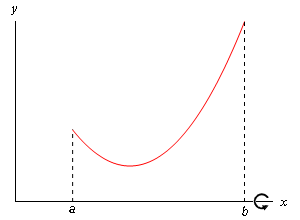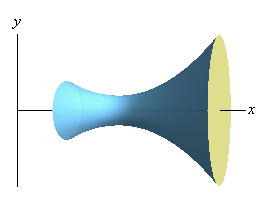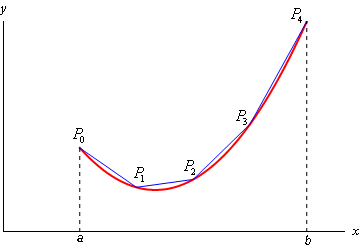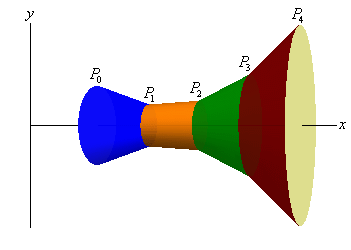Paul's Online Notes
Home / Calculus II / Applications of Integrals / Surface Area
Show Mobile Notice Show All Notes Hide All Notes
Mobile Notice
You appear to be on a device with a "narrow" screen width (i.e. you are probably on a mobile phone). Due to the nature of the mathematics on this site it is best views in landscape mode. If your device is not in landscape mode many of the equations will run off the side of your device (should be able to scroll to see them) and some of the menu items will be cut off due to the narrow screen width.

### Section 8.2 : Surface Area

In this section we are going to look once again at solids of revolution. We first looked at them back in Calculus I when we found the volume of the solid of revolution. In this section we want to find the surface area of this region.

So, for the purposes of the derivation of the formula, let’s look at rotating the continuous function $$y = f\left( x \right)$$ in the interval $$\left[ {a,b} \right]$$ about the $$x$$-axis. We’ll also need to assume that the derivative is continuous on $$\left[ {a,b} \right]$$. Below is a sketch of a function and the solid of revolution we get by rotating the function about the $$x$$-axis.We can derive a formula for the surface area much as we derived the formula for arc length. We’ll start by dividing the interval into $$n$$ equal subintervals of width $$\Delta x$$. On each subinterval we will approximate the function with a straight line that agrees with the function at the endpoints of each interval. Here is a sketch of that for our representative function using $$n = 4$$.Now, rotate the approximations about the $$x$$-axis and we get the following solid.The approximation on each interval gives a distinct portion of the solid and to make this clear each portion is colored differently. Each of these portions are called frustums and we know how to find the surface area of frustums.

The surface area of a frustum is given by,

$A = 2\pi rl$

where,

\begin{align*}r = \frac{1}{2}\left( {{r_1} + {r_2}} \right)\hspace{0.25in}\hspace{0.25in}{r_1} = & {\mbox{radius of right end}}\\ \hspace{0.25in}\hspace{0.25in}\hspace{0.25in}{r_2} = & {\mbox{radius of left end}}\end{align*}

and $$l$$ is the length of the slant of the frustum.

For the frustum on the interval $$\left[ {{x_{i - 1}},{x_i}} \right]$$ we have,

\begin{align*}{r_1} = & f\left( {{x_i}} \right)\\ {r_2} = & f\left( {{x_{i - 1}}} \right)\\ l = & \left| {{P_{i - 1}}\,\,{P_i}} \right|\,\,\,\,\,\,\left( {{\mbox{length of the line segment connecting }}{P_i}{\mbox{ and }}{P_{i - 1}}} \right)\end{align*}

and we know from the previous section that,

$\left| {{P_{i - 1}}\,\,{P_i}} \right| = \sqrt {1 + {{\left[ {f'\left( {x_i^*} \right)} \right]}^2}} \,\,\,\Delta x\,\,\,\,\,{\mbox{where }}x_i^*{\mbox{ is some point in }}\left[ {{x_{i - 1}},{x_i}} \right]$

Before writing down the formula for the surface area we are going to assume that $$\Delta x$$ is “small” and since $$f\left( x \right)$$ is continuous we can then assume that,

$f\left( {{x_i}} \right) \approx f\left( {x_i^*} \right)\hspace{0.25in}\hspace{0.25in}{\mbox{and}}\hspace{0.25in}\hspace{0.25in}f\left( {{x_{i - 1}}} \right) \approx f\left( {x_i^*} \right)$

So, the surface area of the frustum on the interval $$\left[ {{x_{i - 1}},{x_i}} \right]$$ is approximately,

\begin{align*}{A_{\,i}} & = 2\pi \left( {\frac{{f\left( {{x_i}} \right) + f\left( {{x_{i - 1}}} \right)}}{2}} \right)\left| {{P_{i - 1}}\,\,{P_i}} \right|\,\,\\ & \approx 2\pi f\left( {x_i^*} \right)\sqrt {1 + {{\left[ {f'\left( {x_i^*} \right)} \right]}^2}} \,\,\,\Delta x\end{align*}

The surface area of the whole solid is then approximately,

$S \approx \sum\limits_{i = 1}^n {2\pi f\left( {x_i^*} \right)\sqrt {1 + {{\left[ {f'\left( {x_i^*} \right)} \right]}^2}} \,\,\,\Delta x}$

and we can get the exact surface area by taking the limit as $$n$$ goes to infinity.

\begin{align*}S & = \mathop {\lim }\limits_{n \to \infty } \sum\limits_{i = 1}^n {2\pi f\left( {x_i^*} \right)\sqrt {1 + {{\left[ {f'\left( {x_i^*} \right)} \right]}^2}} \,\,\,\Delta x} \\ & = \int_{{\,a}}^{{\,b}}{{2\pi f\left( x \right)\sqrt {1 + {{\left[ {f'\left( x \right)} \right]}^2}} \,dx}}\end{align*}

If we wanted to we could also derive a similar formula for rotating $$x = h\left( y \right)$$ on $$\left[ {c,d} \right]$$ about the $$y$$-axis. This would give the following formula.

$S = \int_{{\,c}}^{{\,d}}{{2\pi \,h\left( y \right)\sqrt {1 + {{\left[ {h'\left( y \right)} \right]}^2}} \,dy}}$

These are not the “standard” formulas however. Notice that the roots in both of these formulas are nothing more than the two $$ds$$’s we used in the previous section. Also, we will replace $$f\left( x \right)$$ with $$y$$ and $$h\left( y \right)$$ with $$x$$. Doing this gives the following two formulas for the surface area.

#### Surface Area Formulas

\begin{array}{ll}\begin{align*}S = \int{{2\pi y\,ds}}\hspace{0.25in}\hspace{0.25in}{\mbox{rotation about }}x - {\mbox{axis}}\\ S = \int{{2\pi x\,ds}}\hspace{0.25in}\hspace{0.25in}{\mbox{rotation about }}y - {\mbox{axis}}\end{align*}\end{array}

where,

\begin{array}{ll}\begin{align*}ds = \sqrt {1 + {{\left( {\frac{{dy}}{{dx}}} \right)}^2}} \,dx\,\hspace{0.25in}{\mbox{if }}y = f\left( x \right),\,\,a \le x \le b\\ ds = \sqrt {1 + {{\left( {\frac{{dx}}{{dy}}} \right)}^2}} \,dy\,\hspace{0.25in}{\mbox{if }}x = h\left( y \right),\,\,c \le y \le d\end{align*}\end{array}

There are a couple of things to note about these formulas. First, notice that the variable in the integral itself is always the opposite variable from the one we’re rotating about. Second, we are allowed to use either $$ds$$ in either formula. This means that there are, in some way, four formulas here. We will choose the $$ds$$ based upon which is the most convenient for a given function and problem.

Now let’s work a couple of examples.

Example 1 Determine the surface area of the solid obtained by rotating $$y = \sqrt {9 - {x^2}}$$, $$- 2 \le x \le 2$$ about the $$x$$-axis.
Show Solution

The formula that we’ll be using here is,

$S = \int{{2\pi y\,ds}}$

since we are rotating about the $$x$$-axis and we’ll use the first $$ds$$ in this case because our function is in the correct form for that $$ds$$ and we won’t gain anything by solving it for $$x$$.

Let’s first get the derivative and the root taken care of.

$\frac{{dy}}{{dx}} = \frac{1}{2}{\left( {9 - {x^2}} \right)^{ - \frac{1}{2}}}\left( { - 2x} \right) = - \frac{x}{{{{\left( {9 - {x^2}} \right)}^{\frac{1}{2}}}}}$ $\sqrt {1 + {{\left( {\frac{{dy}}{{dx}}} \right)}^2}} = \sqrt {1 + \frac{{{x^2}}}{{9 - {x^2}}}} = \sqrt {\frac{9}{{9 - {x^2}}}} = \frac{3}{{\sqrt {9 - {x^2}} }}$

Here’s the integral for the surface area,

$S = \int_{{\, - 2}}^{{\,2}}{{2\pi y\frac{3}{{\sqrt {9 - {x^2}} }}\,dx}}$

There is a problem however. The $$dx$$ means that we shouldn’t have any $$y$$’s in the integral. So, before evaluating the integral we’ll need to substitute in for $$y$$ as well.

The surface area is then,

\begin{align*}S & = \int_{{\, - 2}}^{{\,2}}{{2\pi \sqrt {9 - {x^2}} \frac{3}{{\sqrt {9 - {x^2}} }}\,dx}}\\ & = \int_{{\, - 2}}^{{\,2}}{{6\pi \,dx}}\\ & = 24\pi \end{align*}

Previously we made the comment that we could use either $$ds$$ in the surface area formulas. Let’s work an example in which using either $$ds$$ won’t create integrals that are too difficult to evaluate and so we can check both $$ds$$’s.

Example 2 Determine the surface area of the solid obtained by rotating $$y = \sqrt{x}$$, $$1 \le y \le 2$$ about the $$y$$-axis. Use both $$ds$$’s to compute the surface area.
Show Solution

Note that we’ve been given the function set up for the first $$ds$$ and limits that work for the second $$ds$$.

Solution 1
This solution will use the first $$ds$$ listed above. We’ll start with the derivative and root.

$\frac{{dy}}{{dx}} = \frac{1}{3}{x^{ - \frac{2}{3}}}$ $\sqrt {1 + {{\left( {\frac{{dy}}{{dx}}} \right)}^2}} = \sqrt {1 + \frac{1}{{9{x^{\frac{4}{3}}}}}} = \sqrt {\frac{{9{x^{\frac{4}{3}}} + 1}}{{9{x^{\frac{4}{3}}}}}} = \frac{{\sqrt {9{x^{\frac{4}{3}}} + 1} }}{{3{x^{\frac{2}{3}}}}}$

We’ll also need to get new limits. That isn’t too bad however. All we need to do is plug in the given $$y$$’s into our equation and solve to get that the range of $$x$$’s is $$1 \le x \le 8$$. The integral for the surface area is then,

\begin{align*}S & = \int_{{\,1}}^{{\,8}}{{2\pi x\frac{{\sqrt {9{x^{\frac{4}{3}}} + 1} }}{{3{x^{\frac{2}{3}}}}}\,dx}}\\ & = \frac{{2\pi }}{3}\int_{{\,1}}^{{\,8}}{{{x^{\frac{1}{3}}}\sqrt {9{x^{\frac{4}{3}}} + 1} \,dx}}\end{align*}

Note that this time we didn’t need to substitute in for the $$x$$ as we did in the previous example. In this case we picked up a $$dx$$ from the $$ds$$ and so we don’t need to do a substitution for the $$x$$. In fact, if we had substituted for $$x$$ we would have put $$y$$’s into the integral which would have caused problems.

Using the substitution

$u = 9{x^{\frac{4}{3}}} + 1\hspace{0.25in}\hspace{0.25in}\hspace{0.25in}du = 12{x^{\frac{1}{3}}}\,dx$

the integral becomes,

\begin{align*}S & = \frac{\pi }{{18}}\int_{{\,10}}^{{\,145}}{{\sqrt u \,du}}\\ & = \left. {\frac{\pi }{{27}}{u^{\frac{3}{2}}}} \right|_{10}^{145}\\ & = \frac{\pi }{{27}}\left( {{{145}^{\frac{3}{2}}} - {{10}^{\frac{3}{2}}}} \right) = 199.48\end{align*}

Solution 2
This time we’ll use the second $$ds$$. So, we’ll first need to solve the equation for $$x$$. We’ll also go ahead and get the derivative and root while we’re at it.

$x = {y^3}\hspace{0.25in}\hspace{0.25in}\hspace{0.25in}\frac{{dx}}{{dy}} = 3{y^2}$ $\sqrt {1 + {{\left( {\frac{{dx}}{{dy}}} \right)}^2}} = \sqrt {1 + 9{y^4}}$

The surface area is then,

$S = \int_{{\,1}}^{{\,2}}{{2\pi x\sqrt {1 + 9{y^4}} \,dy}}$

We used the original $$y$$ limits this time because we picked up a $$dy$$ from the $$ds$$. Also note that the presence of the $$dy$$ means that this time, unlike the first solution, we’ll need to substitute in for the $$x$$. Doing that gives,

\begin{align*}S & = \int_{{\,1}}^{{\,2}}{{2\pi {y^3}\sqrt {1 + 9{y^4}} \,dy}}\hspace{0.25in}\hspace{0.25in}u = 1 + 9{y^4}\\ & = \frac{\pi }{{18}}\int_{{\,10}}^{{\,145}}{{\sqrt u \,du}}\\ & = \frac{\pi }{{27}}\left( {{{145}^{\frac{3}{2}}} - {{10}^{\frac{3}{2}}}} \right) = 199.48\end{align*}

Note that after the substitution the integral was identical to the first solution and so the work was skipped.

As this example has shown we can use either $$ds$$ to get the surface area. It is important to point out as well that with one $$ds$$ we had to do a substitution for the $$x$$ and with the other we didn’t. This will always work out that way.

Note as well that in the case of the last example it was just as easy to use either $$ds$$. That often won’t be the case. In many examples only one of the $$ds$$ will be convenient to work with so we’ll always need to determine which $$ds$$ is liable to be the easiest to work with before starting the problem.